# Circuit Enhancement Enables Digital Setting of Voltage Reference

## Texas Instruments LM4041 TLV431 TPL0102

Christopher Dean, Texas Instruments

Electronic Design

By adding a digital potentiometer and ratiometric division to a voltage-reference IC's feedback loop, you can set the reference's output voltage using a digital code.

Adjustable voltage references offer great flexibility to circuit designers because the reference isn’t limited to the manufacturer’s preset values. The adjustable output is typically configured with a voltage divider from the output to the feedback pin (Fig. 1). To regulate the output, the circuit compares the voltage at the feedback pin to an internal reference (shown in this article as VREF_INT), typically 1.2 V. The device adjusts the output until VFB and VREF_INT match.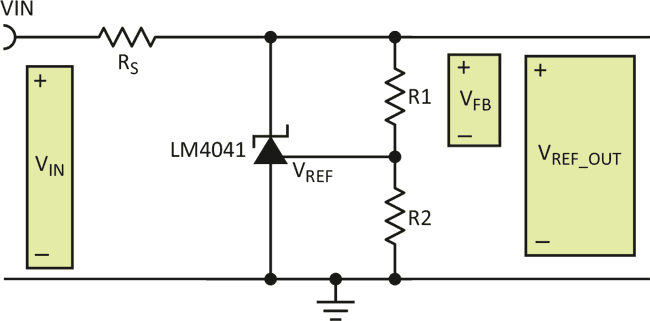Figure 1. In a typical adjustable voltage reference, a voltage divider between the output and feedback pin is used to enable setting of the output value.

Some adjustable shunt references, such as the LM4041-N, maintain VFB across R1; some maintain VFB across R2, such as the TLV431. This circuit enhancement shows one possible method of changing the resistor divider and in turn changing the reference voltage with digital signals. It uses the LM4041-N, but the concepts apply equally to other adjustable shunt references by switching R1 and R2 in the equations.

The method involves replacing the two fixed resistors with a single digital potentiometer. Figure 2 demonstrates the concept, where the feedback pin is connected to the wiper of the potentiometer and the high side and low side are connected to VREF_OUT and GND, respectively. Figure 3 shows the circuit redrawn, with the TPL0102 digital potentiometer as the voltage divider.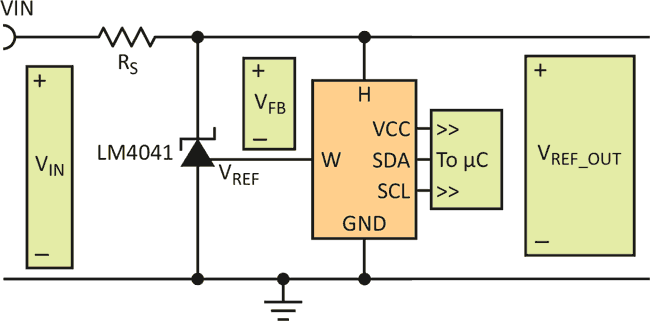Figure 2. By substituting a digital potentiometer for the two fixed resistors, a structure is established for digitally setting the output voltage.

To configure a digital potentiometer to act as a voltage divider, connect a voltage across the high and low pins of the internal resistor and connect the output to the wiper pin. The wiper position affects the ratio of the resistance between the wiper and the high and low pins, and its position is controlled digitally by sending a code word to the device. The TPL0102 uses an I2C interface, while other potentiometers are available with a serial peripheral interface (SPI) or parallel interfaces.

Since the ratio of the resistances sets the output voltage, the absolute values of the divider resistors are not critical. This allows you to easily replace the resistor divider with a digital potentiometer, with this relationship between the adjusted output, VREF_OUT and the resistance ratio: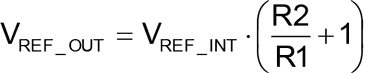(1)

This is an important consideration, as the absolute resistance value of digital potentiometers can vary significantly, but the ratio of the resistances is very accurate. For example, to generate a 3.3-V reference voltage, the required resistance ratio of R2-to-R1 is 1.66.

The potentiometer datasheet provides the formulas to calculate the output of the voltage divider for a given code, shown in Equations 2 and 3. VHW is the voltage from the high pin (H) to the wiper (W), while VWL is the voltage from the wiper (W) to the low pin (L):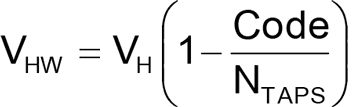(2)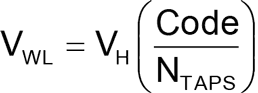(3)

VFB is across R1, so we use Equation 2, which calculates the voltage between the high pin and the wiper pin. The wiper is connected to the feedback pin of the device, and VFB is forced to VREF_INT. Equation 4 shows the formula solved for the digital code required for VREF_OUT: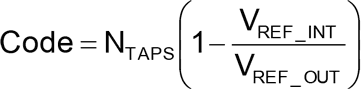(4)

Continuing with this the example in Equation 5, where NTAPS is 256, VREF_INT is 1.24 V and VREF_OUT is 3.3 V, you need to write decimal code 160, which yields resistance values of 37.50 kΩ for R1 and 62.50 kΩ for R2. More importantly, the ratio of these two resistances is 1.66, also calculated using Equation 1: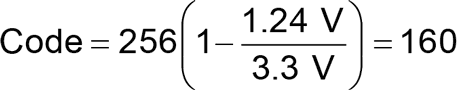(5)

To change the reference voltage, simply write an I2C-bus transaction to move the wiper position accordingly. As a result, the voltage at the feedback pin changes and adjusts VREF_OUT. You can also use the potentiometer to digitally "tune" the reference voltage. A greater number of taps in the potentiometer yields finer resolution in the resistance ratio, and therefore finer resolution in the output-reference voltage.

A limitation of using a digital potentiometer in this application is the voltage limit of the digital potentiometer IC, which typically cannot exceed 5.5 V. Therefore, it's important to confirm that the resistance ratio does not get set to a condition that would present a VREF_OUT greater than 5.5 V. For a 256-tap digital potentiometer and a shunt reference with an internal reference of 1.24 V, the decimal code should not exceed 200. Figure 4 demonstrates the effect of the input code on the reference voltage for a 256-tap potentiometer and a 1.24-V-referenced device.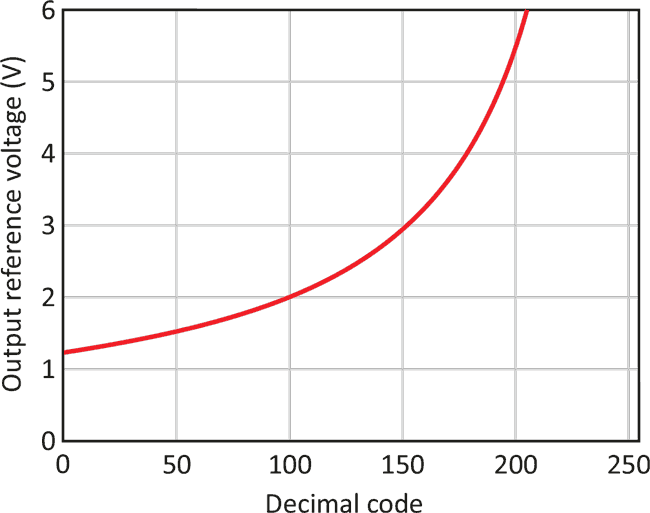Figure 4. The relationship between digital code and reference voltage is not linear, but can easily be calculated as needed, or determined in advance and stored.

You should enable and configure the potentiometer before powering the shunt reference to ensure that the resistor divider is correctly in place. If that’s not possible, add a large resistance in parallel with the resistor that’s not acting as the drop for VFB. That would be 1 MΩ from the feedback pin to ground (across R2) for the LM4041, or 1 MΩ from the feedback pin to the output (across R1) for the TLV431.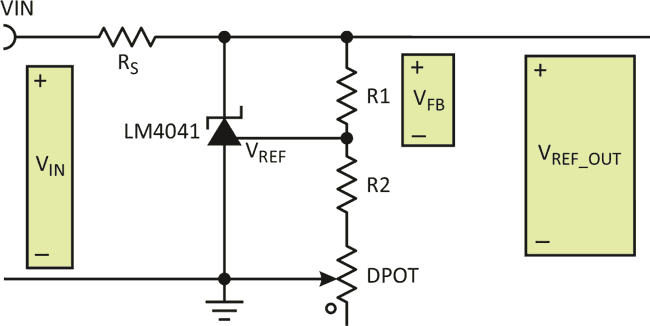Figure 5. Configuring the potentiometer as a rheostat avoids the need for an extra resistance in parallel. However, it now makes the circuit behavior dependent on an absolute resistance value rather than precisely known resistance ratios.

To avoid an extra parallel resistance, configure the device with fixed R1 and R2 resistors and a digital potentiometer in series with one of them. You would then have to configure the potentiometer as a rheostat (Fig. 5). This configuration depends on the absolute resistance of the digital potentiometer, which is not as accurate as when using it as a ratiometric voltage divider – it requires feedback to the microcontroller for final digital-code selection. Technical references 1 through 3 provide additional information on voltage references, their topologies, and their operation.

## Materials on the topic

electronicdesign.com